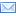#Difference Between Similar Terms and Objects

# Difference Between Inequalities and EquationsInequalities vs Equations

Algebra is a branch of mathematics that is concerned with the study of operations and relations as well as constructions and concepts of equations, terms, and algebraic structures. Its roots can be traced back to the Ancient Babylonians.

They developed formulas to calculate solutions to mathematical problems while early Egyptian, Greek, and Chinese mathematicians solved mathematical problems by using geometric methods.

Later, Arabic and Muslim mathematicians developed sophisticated algebraic methods in solving linear indeterminate equations, quadratic equations, and equations with multiple variables. Today, we solve mathematical problems by using these methods, particularly by using linear equations and inequalities.

An equation is a statement that maintains the equal value of two mathematical expressions. If the statement is true for all variable values, it is called an identity. If it is only true for some variable values, it is called a conditional equation.

An inequality, on the other hand, is a statement that uses the symbols > for greater than or < for lesser than to denote that one quantity is larger or smaller in value than another. Like an identity, an inequality holds values for all variables. It focuses on the inequalities of two variables with one as their exponents. Its graphs include a dashed line that shows if they are greater or lesser than each other or if they are not equal to each other. It is very complex and needs assessment as to how to resolve the additional set of solutions. An equation only involves simple slope and intercept analysis making it less complex. Its graphs include a solid line in all the equations. While a linear equation of two variables can have more than one solution, a linear inequality involves several sets of solutions. An equation shows the equality of two amounts or variables, and it has only one answer to a problem although it can have different solutions. It uses factors such as x, y, etc. An inequality, on the other hand, shows how numbers or variables are ordered, whether they are lesser than, more than, or equal to each other. Examples: Equation: a) x + 10 = 15 , x = 15 ‘“ 10 , x = 5 b) 2x + 20 = 40 , 2x = 40 ‘“ 20 , 2x = 20 x = 20/2 , x = 10 Inequality: a) 10 > 5

b) 2x + 10 > 0 , 2x >10 , x > 10/2 ,

x > 5, which means any value that is more than 5 can be the

solution. In which case, there are several.

Summary:

1. An equation is a mathematical statement that shows the equal value of two expressions while an inequality is a mathematical statement that shows that an expression is lesser than or more than the other.
2. An equation shows the equality of two variables while an inequality shows the inequality of two variables.
3. Although both can have several different solutions, an equation only has one answer while an inequality can also have several.
4. An equation uses factors like x and y while an inequality uses symbols such as < and >.

### Search DifferenceBetween.net :

Custom Search

Help us improve. Rate this post!(6 votes, average: 2.67 out of 5)Loading...Email This Post : If you like this article or our site. Please spread the word. Share it with your friends/family.

1. Equations are algebraic and use x and y. Inequalities use and is also a mathematical statement.

2. Very good

3. It is so bad i would rather die than to read this k

4. hahahahaha i just skipped to the bottom. nobody got time to read all of dat.

5. good get to the point

Please note: comment moderation is enabled and may delay your comment. There is no need to resubmit your comment.

Articles on DifferenceBetween.net are general information, and are not intended to substitute for professional advice. The information is "AS IS", "WITH ALL FAULTS". User assumes all risk of use, damage, or injury. You agree that we have no liability for any damages.